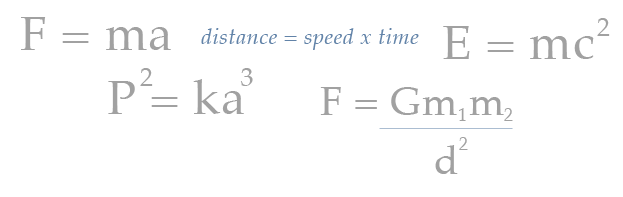# Voyage’s Use of Numerical Models

A Numerical Model uses mathematical equations to represent some reality to be studied. It is the familiarity with mathematics—the language of nature—that allows the student to embrace the equations, test the equations against observable real world variables, and make predictions.

In Voyage’s case, the central numerical model is the equation of scale:

Model Dimension = Real Dimension/10,000,000,000

It is used heavily in the educational materials, allowing students to calculate, on the scale of Voyage, the sizes of the Sun, planets, moons, and other Solar System objects, as well as the speed of a planet orbiting the Sun, the speed and location of spacecraft such as Voyager 1 and 2, and the speed of light. The equation of scale is used by students to create a physical model of the Solar System, and to characterize how such a model would be set in motion.

Another example of a numerical model used in Voyage is a middle school lesson that uses the equation of an ellipse, coupled with known parameters defining the planetary orbits, to create a physical model of orbits in the Solar System (see the diagram to the right). The student then explores the nature of the generated ellipses and sees that the planets orbit the Sun in nearly circular orbits. But the orbits for a comet and a Trans-Neptunian Object have far more elliptical orbits than the planets. This is also the case for Pluto, which provides some evidence that Pluto might be a large Trans-Neptunian Object rather than a small planet.

At the high school level, the apparent size of the Sun in photographs taken at different times of the year is used to generate the equation of Earth’s orbit around the Sun, and a physical model of that orbit.